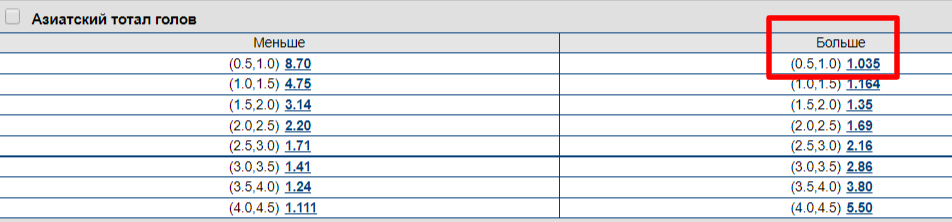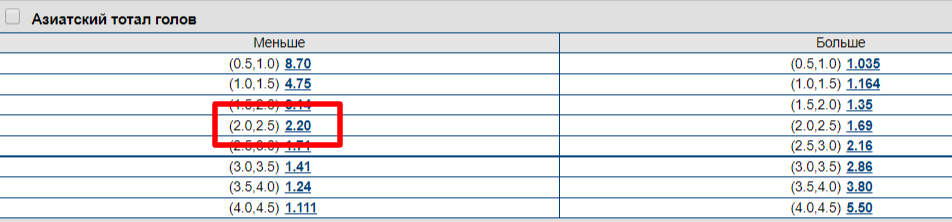# The difference between traditional and Asian total

15.10.2021

For the majority of Russian players, the usual, half totals are much more familiar. TB (1.5) goals, TM (2) goals in football - this is understandable to everyone who has at least minimal experience of interacting with bookmakers. But with Asian totals, everything is a little more complicated, the winnings are calculated differently.

## What is asian total?

Asian totals add intermediate values ​​between traditional totals. It is popular with Asian bookmakers, hence its name. It is also called quarter, because the numerical values ​​of Asian totals are between the traditional half.

For example:

1. Asian TB (0.5: 1) is the average between TB (0.5) and TB (1);
2. Asian TM (2; 2.5) is the average between TM (2) and TM (2.5);
3. Asian TM (1.25) is the average between TM (1) and TM (1.5).

The only confusion lies in the form of writing Asian totals in the line of bookmakers. There are two forms: TB (0.75) and TB (0.5; 1) are the same thing. As well as a pair of TM (3.25) and TM (3; 3.5). The first form is adopted by Asians, the second - in bookmakers in Europe and the CIS. In the second case, both values ​​of the traditional totals are written, and in the first - the average between them.

## How Asian total is calculated?

Here are some examples of calculating Asian total, so that the reader will once and for all understand the principle of calculating this type of bet.1. TB (0.5; 1) or TB (0.75) heads. Half of the bet is placed on TB (0.5), and half on TB (1). If 0 goals are scored in the match, both sides lose. With exactly one goal in the match, half of the bet wins (with odds of 1.035), the second half of the bet amount is returned. With two or more goals, both halves of the bet win. In the latter case, the winnings are calculated using the odds of 1.035.1. TM (2; 2.5) or TM (2.25) heads. Half of the bet goes to TM (2), and half to TM (2.5). If there are three or more goals in the match, the entire bet is lost. If two goals are scored in the match, half of the bet wins (with odds of 2.20), the second half of the bet amount is refunded. If there are zero or one goals, the entire bet (both halves) wins. The winnings are calculated using the odds of 2.20.

***

In general, everything is simple. An intermediate value is added between the figures of the traditional total, and the bet is divided in half between the adjacent traditional totals. Each half is calculated separately, including a separate settlement in both express and express systems.

***

Asian totals add variability to betting bets, especially in low-performing sports. If you are in doubt between TB (2) and TB (2.5) - the rate on TB (2.25) will increase the coefficient on the first outcome, and reduce the risks relative to the second value.